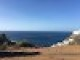(15 votes, average: 5.00 out of 5)Loading...

## Project Euler 70 Solution## Project Euler 70: Investigate values of n for which φ(n) is a permutation of n.

#### Project Euler 70 Problem Description

Euler’s Totient function, φ(n) [sometimes called the phi function], is used to determine the number of positive numbers less than or equal to n which are relatively prime to n. For example, as 1, 2, 4, 5, 7, and 8, are all less than nine and relatively prime to nine, φ(9)=6.
The number 1 is considered to be relatively prime to every positive number, so φ(1)=1.

Interestingly, φ(87109)=79180, and it can be seen that 87109 is a permutation of 79180.

Find the value of n, 1 <n < 107, for which φ(n) is a permutation of n and the ratio n/φ(n) produces a minimum.

#### Analysis

We can reduce our search significantly by selecting prime pairs (p1, p2 ) and calculate n as n = p1 x p2. This allows the totient to be calculated as φ(n) = (p1-1)(p2-1) for n. Now, just find the minimum ratio n/φ(n) for those n and φ(n) that are permutations of one another.

The range of primes was selected by taking the square root of the upper bound, 10,000,000, which is about 3162 and taking ±30% for a range of primes from 2000 to 4000 (247 primes).

#### Project Euler 70 Solution

Runs < 0.001 seconds in Python 2.7.Use this link to get the Project Euler 70 Solution Python 2.7 source.

#### Afterthoughts

Project Euler 70 Solution last updated

## Discussion

### 12 Responses to “Project Euler 70 Solution”

1.Since this is from almost 10 years ago I doubt I’ll get an answer but can you please explain why you are doing this:
if (p1+p2)%9 != 1:

Thanks for you site it has been much help for a guy that haven’t done any math for the last 20 years

Posted by Yo | November 20, 2018, 10:58 PM
•He mentioned this in the footnotes. It make sense after you see what’s going one. Hope this helps.

The line: if(p1+p2)%9 != 1: continue is an optimization that observes for two integers to be permutations of one another their difference must be a multiple of 9.

Posted by MarkM | December 4, 2018, 12:11 AM
2.Hi,
I was slightly confused about the algorithm used here and few questions about it.
1. You have only taken in num as p1*p2 . but the number could be (p1**k1) * (p2 ** k2).
How did you eliminate that they will only be product of two primes not product of higher powers.
2. Also how you came to conclusion about the number of primes to in +- 30% of square_root.
Thanks
Ayushman
P.s:- Thank a lot for posting the algorithm.

Posted by ayushman | June 18, 2012, 12:13 AM
3.I don’t understand how you know n is necessarily the product of two primes. Can you please explain that to me?

Thanks

Posted by Michael | April 27, 2010, 2:47 AM
•This solution was written a long time ago using Perl which can be difficult to follow if you’re not familar with the language.

‘\$n’ is the product of two primes because we are using primes read into an array ‘@p’. The list of primes is appended to the end of the program.

The statement:
@p = split /s/,;
reads all the prime numbers into the array at once.

Posted by Mike | April 27, 2010, 10:41 AM
•Yes, I understood that part, but what I was asking was how you limited n to be the product of two primes. Why can’t n have three or more prime factors?

Posted by Michael | April 27, 2010, 10:50 AM
•That is because the problem is asking for the minimal ratio:
We have prime pairs (p1, p2 ) and calculate n as n = p1 x p2. Let’s assume n’ is a product of prime tuple (p1, p2, p3). r is ratio of n/φ(n), r’ is ratio of n’/φ(n’). So r/r’ = (p3-1)/p3 which is smaller than 1. r < r', so a composite number n which is a product of two primes will produce the minimal ratio

Posted by Yang Yang | December 30, 2010, 5:25 AM
•I think Yang Yang explained it best. Thanks for your contribution.

Posted by Mike | December 31, 2010, 12:56 AM
4.This seems to work fine and we tested it against our (much slower) solution for 10^10 but having to feed it primes is a pain. I would also would like to know more about the 25% thing. It works but not sure why.

Thanks for the great solutions and help with the problems. This is by far the best site I’ve found.

Posted by Tarren | May 25, 2009, 10:14 AM
5.I have a question, I am not a math guru, but why and how did you find it to be the square root +-25% for the range of primes, do you have somewhere I can read to see the proof for that. I am just curious, and was baffled with this problem until I found your solution.

Thanks!

Posted by Steven Carnes | May 22, 2009, 10:39 AM
•This is a question for Christner to whom I have forwarded your enquiry.

Posted by Mike | May 22, 2009, 2:25 PM